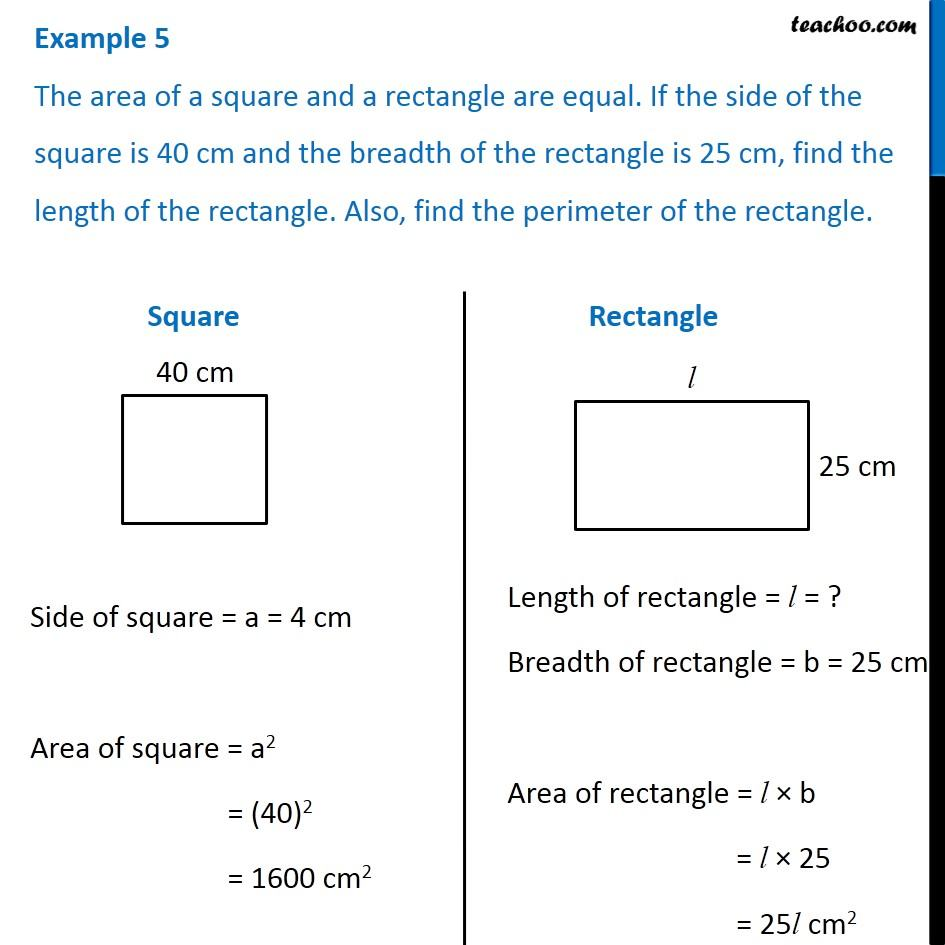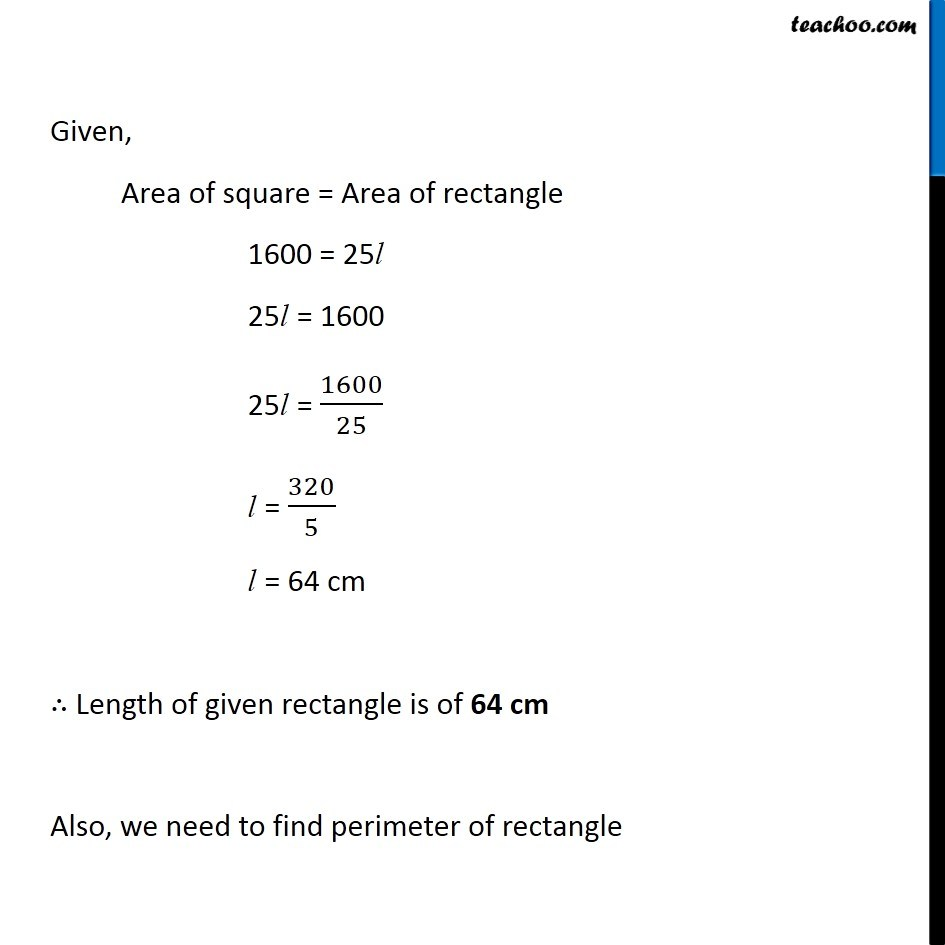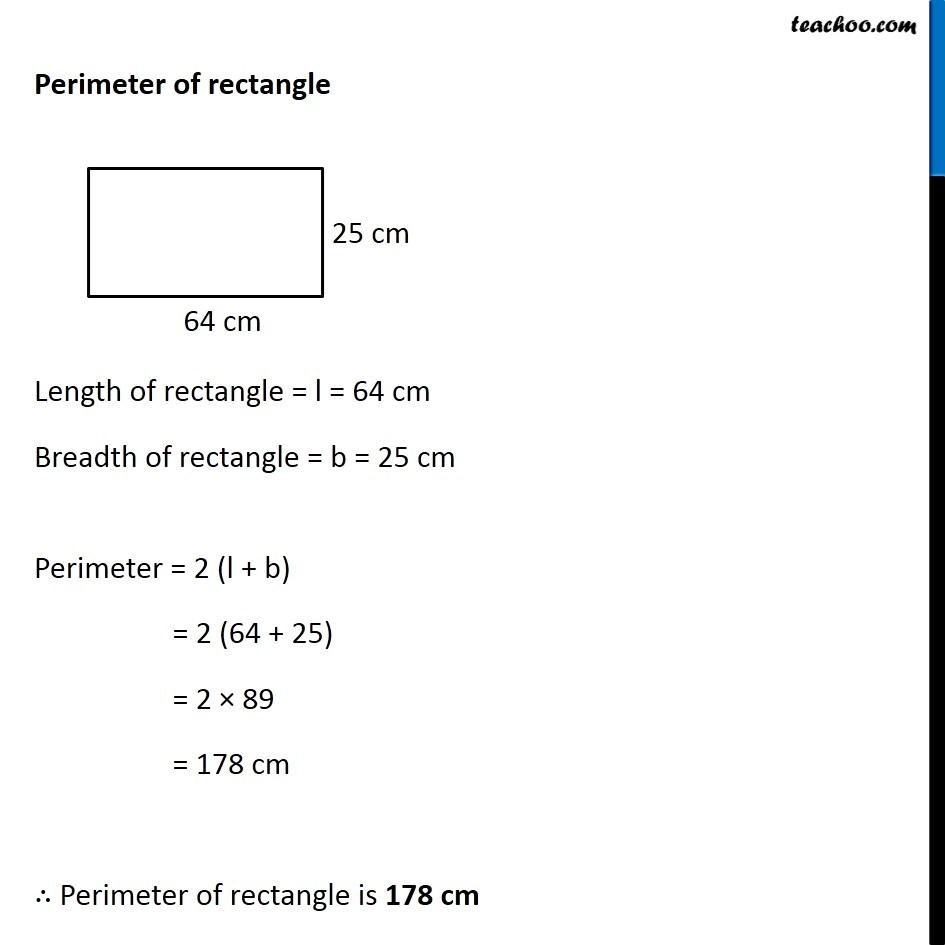Examples

Chapter 9 Class 7 Perimeter and Area
Serial order wiseLearn in your speed, with individual attention - Teachoo Maths 1-on-1 Class

### Transcript

Question 5 The area of a square and a rectangle are equal. If the side of the square is 40 cm and the breadth of the rectangle is 25 cm, find the length of the rectangle. Also, find the perimeter of the rectangle. Square Side of square = a = 4 cm Area of square = a2 = (40)2 = 1600 cm2 Rectangle Length of rectangle = l = ? Breadth of rectangle = b = 25 cm Area of rectangle = l × b = l × 25 = 25l cm2 Given, Area of square = Area of rectangle 1600 = 25l 25l = 1600 25l = 1600/25 l = 320/5 l = 64 cm ∴ Length of given rectangle is of 64 cm Also, we need to find perimeter of rectangle Perimeter of rectangle Length of rectangle = l = 64 cm Breadth of rectangle = b = 25 cm Perimeter = 2 (l + b) = 2 (64 + 25) = 2 × 89 = 178 cm ∴ Perimeter of rectangle is 178 cm